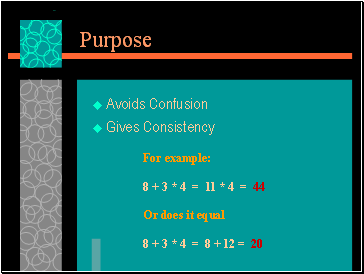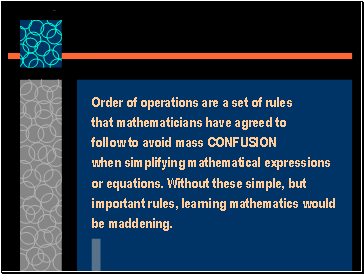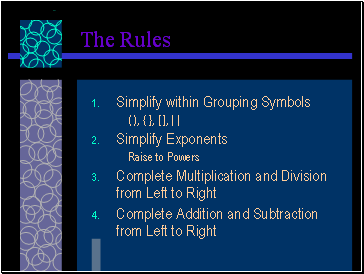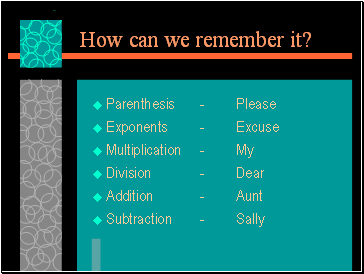# Order of OperationsPage 1

#### WATCH ALL SLIDES

Slide 1## Order of Operations

Mr. Clutter

VMS Library

Slide 2Order of Operations

A standard way to simplify mathematical expressions and equations.

Slide 3## Purpose

Avoids Confusion

Gives Consistency

For example:

8 + 3 * 4 = 11 * 4 = 44

Or does it equal

8 + 3 * 4 = 8 + 12 = 20

Slide 4Order of operations are a set of rules

that mathematicians have agreed to

when simplifying mathematical expressions

or equations. Without these simple, but

important rules, learning mathematics would

Slide 5## The Rules

Simplify within Grouping Symbols

( ), { }, [ ], | |

Simplify Exponents

Raise to Powers

Complete Multiplication and Division from Left to Right

Complete Addition and Subtraction from Left to Right

Slide 6## Back to Our Example

For example:

8 + 3 * 4 = 11 * 4 = 44

Or does it equal

8 + 3 * 4 = 8 + 12 = 20

Using order of operations, we do the

multiplication first. So what’s our

20

Slide 7## How can we remember it?

Exponents - Excuse

Multiplication - My

Division - Dear

Subtraction - Sally

Slide 8## Summary

Key points to remember that will give audience confidence or improve morale